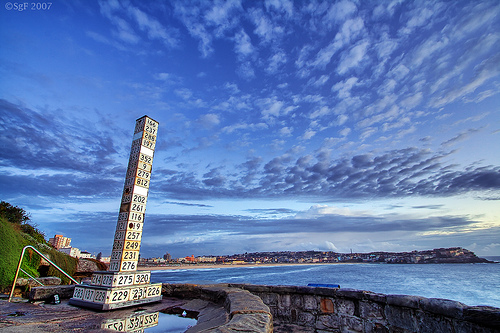Entertainment

# Puzzlin’!Written by

Puzzle 1
* Think of a number.
* Subtract 2.
* Multiply by 4.
* Subtract 2.

Try it with a friend. Because whichever number she/he chooses, the answer will be 18!

Puzzle 2
* Think of a 3-digit number, say 234.
* Reverse that number. That is 432.
* Subtract the smaller number from the larger. 432 – 234 = 198.
* Reverse the number you have got. You get 891.
* Add the last two numbers. That is 198 + 891 = 1089.

Again, try this with a friend. Because whichever initial number she/he chooses, the final answer will always be 1089!

Visual Courtesy:https://www.flickr.com/photos/sacharules/